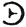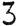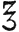Towler, John. The Silver Sunbeam. Joseph H. Ladd, New York: 1864. Electronic edition prepared from facsimile edition of Morgan and Morgan, Inc., Hastings-on-Hudson, New York. Second printing, Feb. 1974. ISBN 871000-005-9

## Chapter XLV. WEIGHTS AND MEASURES.

WEIGHTS and capacities in England and France are estimated from certain standard linear measurements. In England, a pendulum vibrating seconds of time in a vacuum, at the latitude of London, and at the level of the sea, is assumed as the standard of linear dimensions; it is 39.1393 inches. This is the standard, too, of all our measurement of length, capacity, and weight in the United States. But, like the English, we retain all the old and arbitrary systems of weights and measures; whereas the French have assumed. a decimal system in all their measurements that merits the highest praise and imitation. The linear standard of the French, from which they derive all other measurements, is called a métre. It is the ten-millionth part of a quarter of the earth's meridian, and measures 39.371 inches. The métre is divisible decimally in both directions.

The connecting link between the English linear unit and their measures of capacity and weight are as follows:

A cubic inch of distilled water weighed in air with brass weights at a temperature of 62° Fahr., the barometer standing at 30 inches, is equal to two hundred and fifty-two grains and four hundred and fifty-eight one thousandth parts of a grain; of such grains 5760 are required to make the imperial standard troy or apothecaries; pound; and 7000 of such grains make the commercial or avoirdupois pound. The imperial gallon has a capacity of 277.274 cubic inches; and a gallon of distilled water, as above, weighs 10 pounds avoirdupois, or 70,000 grains.

The connecting link between the French linear unit and their measures of weight and capacity, are as follows

A cubic centimétre of distilled water, at its maximum density, at the temperature of 39.5° Fahrenheit, is the unit of weights and is called a gramme, which is divided decimally above and below.

A cubic decimétre is called a litre, which is the unit of the measures of capacity, and divisible decimally.

### Comparison of Weights and Measures. Apothecaries' Weight.

 French gramme. 1 grain = 0.0647 20 grains = 1 scruple = 1.295 60 grains = 3 scruples = 1 drachm = 3.885 480 grains = 8 drachms = 1 ounce = 31.08 5760 grains = 12 ounces = 1 pound = 372.96

### Symbols.

grain=gr. scruple=. drachm=. ounce=. pound=lb

### Apothecaries' Measure of Capacity. (United States.)

 60 minims = 1 fluid drachm 480 minims = 8 fluid drachms = 1 fluid ounce. 7680 minims = 16 fluid ounces = 1 pint. 61,440 minims = 8 pints = 1 gallon.

### Avoirdupois Weight.

 French gramme. 1 drachm = 1.77 16 drachms = 1 ounce = 28.328 16 ounces = 1 pound = 453.25 112 pounds = 1 hundred weight 20 hundred weight = 1 ton

### Apothecaries' grains.

 1 drachm = 27.3438 1 ounce = 437.5 1 pound = 7000 1 hundred weight = 784000 1 ton. = 1.568e+07

 Apothecaries' ounce = 480 grains Avoirdupois ounce = 437.5 grains United States pint = 16 fluid ounces Imperial or British pint 20 fluid ounces United States gallon = 128 fluid ounces = 8 pounds avoirdupois. Imperial or British gallon = 160 fluid ounces = 10 pounds avoirdupois

### Weight of Water at 62° and Capacity of:

 Cubic inches.. Grains 1 gallon (Imperial) = 277.274 = 70000. 1 gallon (U. S.) = 231.000 = 56000. 1 quart (Imperial) = 69.318 = 17500. 1 quart (U. S.) = 57.750 = 14000 1 pint (Imperial) = 34.659 = 8750 1 pint (U. S.) = 28.875 = 7000. 16 fluid ounces = 28.875 = 7000. 1 fluid ounce = 1.732 = 437.5 1 fluid drachm = 0.216 = 54.7 1 minim = 0.0336 = 0.91

### French Measures of Length.

 English. inches. Millimétre = .03937 Centimètre = .39371 Decimétre = 3.93708 Métre = 39.37079 Décamétre = 393.70788 Hectométre = 3937.0788 Kilométre = 39370.788 Myriamétre = 393707.88

### French Weights

 Equivalents in Grains. Milligramme, .0154 Centigramme, .1543 Decigramme, 1.5434 Gramme, 15.434 Decagramme, 154.340 Hectogramme, 1543.402 Kilogramme, 15434.023 Myriogramme, 154340.234

A gramme of water=1 cubic centimétre=15.43 grains=17 minims.

1000 grammes of water=1 litre=1 kilogramme=15434.023 grains=2 lb. 3.27 oz.

 French Liquid Measures. Cubic inches. United States Liquid Measures. Millilitre, .0610 16.2318 minims. Centilitre, .6103 2.7052 fl. drachms. Decilitre, 6.1028 3.3816 fl. ounces. Litre, 61.028 2.1135 pints. Decalitre, 610.280 2.6419 gallons. Hectolitre, 6102.80 26.4190 gallons Kilolitre, 61028.0 264.1900 gallons Myrialitre, 610280 2641.9000 gallons